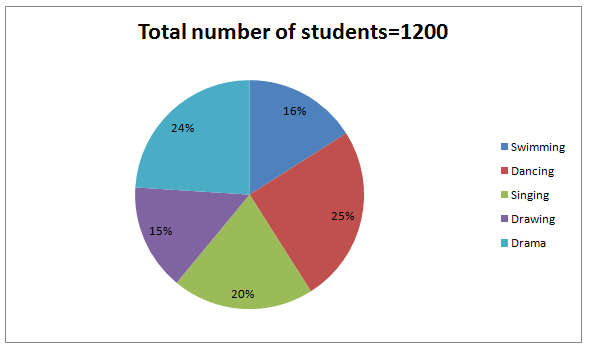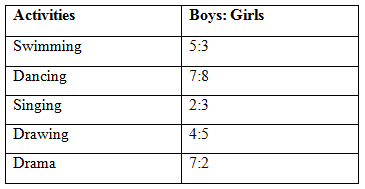# SBI PO Prelims Quantitative Aptitude (Day-20)

Dear Aspirants, Our IBPS Guide team is providing new series of Quantitative Aptitude Questions for SBI PO 2020 so the aspirants can practice it on a daily basis. These questions are framed by our skilled experts after understanding your needs thoroughly. Aspirants can practice these new series questions daily to familiarize with the exact exam pattern and make your preparation effective.

Start Quiz

Ensure Your Ability Before the ExamTake SBI PO 2020 Prelims Free Mock            Test

Data Interpretation

Direction (01-05): Study the following information carefully and answer the given questions:

The given pie chart shows the percentage of students enrolled in different activities in the school.The given table shows the ratio of the number of boys to girls enrolled in these activities.1) Quantity I: Number of girls in the school.

Quantity II: Number of boys in the school.

A) Quantity I > Quantity II

B) Quantity I ≥ Quantity II

C) Quantity II > Quantity I

D) Quantity II ≥ Quantity I

E) Quantity I = Quantity II or Relation cannot be established

2) The boys enrolled in singing and drama is approximately what percent of the number of girls enrolled in Dance and drawing?

A) 123%

B) 138%

C) 113%

D) 103%

E) None of these

3) What is the ratio of the number of girls enrolled in Swimming and Drama together to the number of boys enrolled in Dance and Drawing together?

A) 32:57

B) 34:55

C) 31:51

D) 37:57

E) None of these

4) What is the percentage of girls in the school?

A) 45%

B) 55%

C) 48%

D) 52%

E) None of these

5) The number of girls enrolled in Dance, Drawing and Singing together is approximately what percent of the number of boys in the school?

A) 61%

B) 59%

C) 67%

D) 73%

E) None of these

Application Sums

6) The ratio of the speed of train A and train B is 3:2. Train A crosses the platform of equal length in 54 seconds and train B crosses the pole in 18 seconds. Length of train A is what percent more than that of the length of train B?

A) 110%

B) 125%

C) 130%

D) Cannot be determine

E) None of these

7) Rahul invested certain amount in scheme A which offers simple interest and the amount becomes three-two of itself after 10 years. He also invested Rs.12000 in scheme B which offers compound interest and got Rs.1996.8 after 2 years. What is the difference between the rate of interest in scheme A and the rate of interest in scheme B?

A) 3%

B) 4%

C) 5%

D) 2%

E) None of these

8) The ratio of the speed of stream to speed of the boat in still water is 2:7. If the boat travels against the current at 20kmph, then how much time does it takes to travel 36km downstream?

A) 2 hours

B) 1 hours

C) 54 minutes

D) 48 minutes

E) None of these

9) The average weight of the students in the class is 32kg. 5 new students whose average weight is 28kg joined the class and then average weight of the class becomes 30kg. Find the total number of students in the class?

A) 10

B) 20

C) 15

D) 8

E) None of these

10) The ratio of the ages of A and B is 3:2 and the ratio of ages of B and C after 4 years is 1:2. The present age of A is 60% of the present age of C. Find the present age of B?

A) 12 years

B) 16 years

C) 20 years

D) 8 years

E) None of these

Directions (01 – 05):

Swimming:

Girls = (3/8) * (16/100) * 1200 = 72

Boys = (5/8) *(16/100) * 1200=120

Dance:

Girls = (8/15) * (25/100) * 1200 = 160

Boys =(7/15) * (25/100) * 1200 = 140

Singing:

Girls = (3/5) * (20/100) * 1200 = 144

Boys = (2/5) * (20/100) * 1200= 96

Drawing:

Girls = (5/9) * (15/100) * 1200=100

Boys = (4/9) * (15/100) * 1200 = 80

Drama:

Girls = (2/9) * (24/100) * 1200 = 64

Boys = (7/9) * (24/100) * 1200 = 224

From quantity I,

Number of girls = 72+160+144+100+64=540

From quantity II,

Number of boys = 120+140+96+80+224=660

So, Quantity II > Quantity I

Boys enrolled in singing and drama = 96+224=320

Girls enrolled in Dance and drawing = 100+160=260

Required percentage =(320/260) * 100 = 123%

Required ratio = (72+64) : (140+80)

=136:220 = 34:55

Number of girls = 72+160+144+100+64=540

Required percentage = (540/1200) * 100 = 45%

Number of boys = 120+140+96+80+224 = 660

Required percentage = ((160+100+144)/660) * 100 = 61%

Directions (6-10) :

Let ,

Length of train A=x

Length of train B=y

The speed of train A and train B = 3S : 2S

Distance = speed * time

2x = 3S * (5/18) * 54

x = 45S/2

y = 2S *(5/18) * 18

y= 10S

Required percentage =((45S/2-10S) / 10S)*100

= (25S / 20S) * 100=125%

Scheme A

Consider the principle as P and rate of interest as R,

(3/2) P – P = P*10*R/100

P/2 = PR/10

R=5%

Scheme B

1996.8=12000 * (1+R/100)– 12000

13996.8= (6/5) * (100+R)2

108 = (100+R)

R = 8%

Required Difference = 8-5=3%

Speed of the boat in still water:  speed of stream= 2 : 7

Upstream speed = speed of the boat in still water – speed of stream = 20kmph

⇒ 5x = 20kmph

⇒ x = 4 kmph

Speed of stream= 8kmph

Speed of the boat in still water=28 kmph

Downstream speed= speed of the boat in still water + speed of stream

⇒ 28+8=36kmph

Required time = 36/36 =1 hoursRatio of old students to new student = 2 : 2 = 1 : 1

Total number of students = (5/1) * 2=10

The ratio of the ages of A and B = 3:2

A= (60/100) * C

A= (3/5) * C

((3/5) * C) / B = 3/2

C/B = 5/2

(5x+4) / (2x+4) = 2/1

5x+4 = 4x+8

x= 4

B’s present age = 2(4)= 8 years

 Check Here to View SBI PO Prelims 2020 Quantitative Aptitude Questions Day – 19 Day – 18 Day – 17 Click Here for SBI Clerk 2020 – Detailed Exam Notification